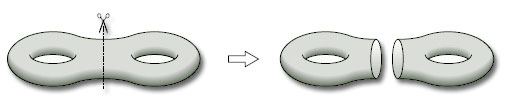Surfaces

Start this free course now. Just create an account and sign in. Enrol and complete the course for a free statement of participation or digital badge if available.

Free course

# 4.5.2 n-fold toruses

We can use a similar technique to find the Euler characteristic of a 2-fold torus. If we cut the surface into two, as shown in Figure 95, and separate the pieces, we obtain two copies of a 1-fold torus with 1 hole, each with Euler characteristic −1.Figure 95

Consider any subdivision of the left-hand torus with 1 hole, and give the right-hand torus with 1 hole its mirror image subdivision. In this way, the vertices and edges on the boundary of each hole can be identified when we glue the two pieces back together. Note that the number of vertices on each boundary must be the same as the number of edges on that boundary.

Thus the vertices and edges on the boundaries contribute a value 0 to the Euler characteristic VE + F of each piece, and also to the Euler characteristic of the 2-fold torus obtained by gluing the two pieces back together. Thus the Euler characteristic of the 2-fold torus is simply the sum of the Euler characteristics of the two pieces: (−1) + (−1) = −2.

We can carry out a similar procedure for a 3-fold torus. If we cut it into two pieces, as shown in Figure 96, we obtain a 2-fold torus with 1 hole and a 1-fold torus with 1 hole. The Euler characteristic of a 2-fold torus with 1 hole is (−2) − 1 = −3, by Theorem 10, and the Euler characteristic of a 1-fold torus with 1 hole is −1, so the Euler characteristic of a 3-fold torus is (−3) + (−1) = −4.

Continuing in this way, we obtain the Euler characteristic of an n-fold torus.

## Theorem 12

The Euler characteristic of an n-fold torus is 2 − 2n.

We can also deduce the following theorem from the above discussion.

## Theorem 13

The Euler characteristic of a surface obtained by gluing together two surfaces with boundary along a given boundary component of each is equal to the sum of the Euler characteristics of the original surfaces.

## Problem 23

Find the Euler characteristic of the surface obtained by gluing a cylinder to a sphere with two holes, where each boundary of the cylinder is glued to the boundary of a separate hole.

A cylinder has Euler characteristic 0 and a sphere with two holes has Euler characteristic 2 − 2 = 0 (by Theorem 11). Therefore, by Theorem 13, the surface obtained by gluing these two surfaces together along the boundaries has Euler characteristic 0 + 0 = 0.

M338_1